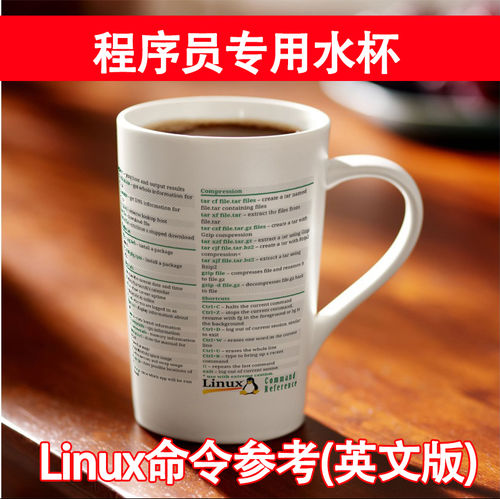JavaScript中有许多章节是关于 `scope` 的,但是对于初学者来说(甚至是一些有经验的JavaScript开发者),这些有关作用域的章节既不直接也不容易理解.

• 什么是作用域?
• 什么是全局(局部)作用域?
• 什么是命名空间,它和作用域有什么不同?
• `this` 关键字是什么,作用于又是怎么影响它的?
• 什么是函数/词法作用域?
• 什么是闭包?
• 什么是共有/私有作用域?
• 我怎么样才能够理解/创建/实践上面所有的情况

### 什么是全局作用域

``````// global scope
var name = 'Todd';``````

``jQuery('.myClass');``

### 什么是局部作用域

``````// Scope A: Global scope out here
var myFunction = function () {
// Scope B: Local scope in here
};``````

``````var myFunction = function () {
var name = 'Todd';
console.log(name); // Todd
};
// Uncaught ReferenceError: name is not defined
console.log(name);``````

### 函数作用域

JavaScript中所有的作用域在创建的时候都只伴随着 `函数作用域` ,循环语句像 `for` 或者 `while` ,条件语句像 `if` 或者 `switch` 都不能够产生新的作用域. `新的函数 = 新的作用域` 这就是规则.下面一个简单的例子用来解释作用域的创建:

``````// Scope A
var myFunction = function () {
// Scope B
var myOtherFunction = function () {
// Scope C
};
};``````

### 词法作用域

``````// Scope A
var myFunction = function () {
// Scope B
var name = 'Todd'; // defined in Scope B
var myOtherFunction = function () {
// Scope C: `name` is accessible here!
};
};``````

``````var myFunction = function () {
var name = 'Todd';
var myOtherFunction = function () {
console.log('My name is ' + name);
};
console.log(name);
myOtherFunction(); // call function
};

// Will then log out:
// `Todd`
// `My name is Todd```````

``````var name = 'Todd';
var scope1 = function () {
// name is available here
var scope2 = function () {
// name is available here too
var scope3 = function () {
// name is also available here!
};
};
};``````

``````// name = undefined
var scope1 = function () {
// name = undefined
var scope2 = function () {
// name = undefined
var scope3 = function () {
var name = 'Todd'; // locally scoped
};
};
};``````

### 闭包

``````var sayHello = function (name) {
var text = 'Hello, ' + name;
return function () {
console.log(text);
};
};``````

``sayHello('Todd'); // nothing happens, no errors, just silence...``

``````var helloTodd = sayHello('Todd');
helloTodd(); // will call the closure and log 'Hello, Todd'``````

``sayHello('Bob')(); // calls the returned function without assignment``

AngularJS的 `\$compile` 方法使用了上面的技术,你可以将当前作用的引用域传递给这个闭包:

``\$compile(template)(scope);``

``````var \$compile = function (template) {
// some magic stuff here
// scope is out of scope, though...
return function (scope) {
};
};``````

### 作用域和 `this`

``````var myFunction = function () {
console.log(this); // this = global, [object Window]
};
myFunction();

var myObject = {};
myObject.myMethod = function () {
console.log(this); // this = Object { myObject }
};

var nav = document.querySelector('.nav'); // <nav class="nav">
var toggleNav = function () {
console.log(this); // this = <nav> element
};

``````var nav = document.querySelector('.nav'); // <nav class="nav">
var toggleNav = function () {
console.log(this); // <nav> element
setTimeout(function () {
console.log(this); // [object Window]
}, 1000);
};

``````var nav = document.querySelector('.nav'); // <nav class="nav">
var toggleNav = function () {
var that = this;
console.log(that); // <nav> element
setTimeout(function () {
console.log(that); // <nav> element
}, 1000);
};

### 使用 `.call()` , `.apply()` 或者 `.bind()`

``````var links = document.querySelectorAll('nav li');
for (var i = 0; i < links.length; i++) {
console.log(this); // [object Window]
}``````

#### .call()和.apply()

`.call()``.apply()` 函数是非常实用的,它们允许你传递一个作用域到一个函数里面,这个作用与绑定了正确的 `this` 值.让我们来处理上面的那些代码吧,让循环里面的 `this` 指向正确的元素值:

``````var links = document.querySelectorAll('nav li');
for (var i = 0; i < links.length; i++) {
(function () {
console.log(this);
}``````

``myFunction(); // invoke myFunction``

``myFunction.call(scope); // invoke myFunction using .call()``

#### .bind()

``````// works

// will invoke the function immediately

``````nav.addEventListener('click', function () {
toggleNav(arg1, arg2);
}, false);``````

``nav.addEventListener('click', toggleNav.bind(scope, arg1, arg2), false);``

### 私有/共有的作用域

``````(function () {
// private scope inside here
})();``````

``````(function () {
var myFunction = function () {
// do some stuff here
};
})();``````

``````(function () {
var myFunction = function () {
// do some stuff here
};
})();

myFunction(); // Uncaught ReferenceError: myFunction is not defined``````

``````// define module
var Module = (function () {
return {
myMethod: function () {
console.log('myMethod has been called.');
}
};
})();

// call module + methods
Module.myMethod();``````

``````// define module
var Module = (function () {
return {
myMethod: function () {

},
someOtherMethod: function () {

}
};
})();

// call module + methods
Module.myMethod();
Module.someOtherMethod();``````

``````var Module = (function () {
var privateMethod = function () {

};
return {
publicMethod: function () {

}
};
})();``````

``````var Module = (function () {
var privateMethod = function () {

};
return {
publicMethod: function () {
// privateMethod();
}
};
})();``````

``````var Module = (function () {
var myModule = {};
var privateMethod = function () {

};
myModule.publicMethod = function () {

};
myModule.anotherPublicMethod = function () {

};
return myModule; // returns the Object with public methods
})();

// usage
Module.publicMethod();``````

``````var Module = (function () {
var _privateMethod = function () {

};
var publicMethod = function () {

};
})();``````

``````var Module = (function () {
var _privateMethod = function () {

};
var publicMethod = function () {

};
return {
publicMethod: publicMethod,
anotherPublicMethod: anotherPublicMethod
}
})();``````